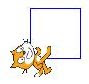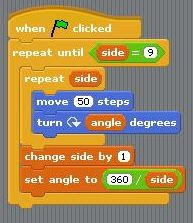## Sunday, February 03, 2008

### Scratch: drawing polygons using variables

Information: the turning angle (external angle) for a polygon = 360 / (num of sides)

1) Draw a squareInitially setup so the cat draws a square of 50 steps, starting initially facing upwards

Point in direction 0 (up)

repeat 4
move 50 steps
turn 90 degrees2) Create two variables: side and angle

Set the angle to 360 / side

Insert the side and angle variables into the polygon procedure as shown:Use the repeat procedure to draw some different shapes (triangle is shown)3) Use the Number, Variable and Control blocks to setup a repeat until, as shown:Now insert the procedure inside the repeat until:Now draw all the shapes at once, 3 to 8 sides: